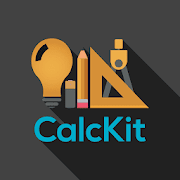# CalcKit : All-In-One Calculator & Unit ConverterEveryone
9,897
·
Offers in-app purchases
CalcKit: All-In-One Calculator is a simple and powerful collection of calculators and converters for mathematics, electronics, finance and more. And best of all, it's completely FREE!

THE PERFECT CALCULATOR

Designed with simplicity in mind, CalcKit helps you solve all kinds of everyday problems.
From simple calculations to unit and currency conversions, percentages, proportions, areas, volumes, etc… it does it all.

Featuring over 150 Calculators and Unit Converters, packed in with a highly customizable Scientific Calculator, CalcKit is the only calculator app you will need on your device.

SCIENTIFIC CALCULATOR
★ Instant results of your calculations as you enter them
★ Simple and full keypad layout
★ Copy/Paste with a single button press
★ Freely movable cursor to modify incorrectly entered expressions
★ Advanced calculator history, able to retrieve both the result and expression of your calculation
★ Floating calculator widget to use the calculator floating over other applications on your phone

150 CALCULATORS & CONVERTERS
★ Algebra, Geometry, Electronics, Finance, Unit Converters & Misc.
★ Currency converter with 160 currencies, available offline
★ Instant results delivered as you type
★ Smart search and sort for faster navigation
★ Create shortcuts on the home screen

CUSTOM CALCULATORS
★ In addition to the many built-in calculators, with CalcKit you can easily create your own calculators and converters in a matter of minutes

★ CalcKit also features a built-in notepad that allows you to easily take notes on the go

List of calculators and converters:

Algebra
• Percentage calculator
• Proportion calculator
• Average calculator - arithmetic, geometric and harmonic mean
• Equation solver - linear, quadratic, cubic and equation system
• Combinations and permutations
• Decimal to fraction
• Fraction simplifier
• Prime number checker
• GCF / LCM calculator - greatest common factor and least common multiple
• Matrix calculator

Geometry
• Triangle, right triangle, square, rectangle, parallelogram, rhombus, trapezoid, hexagon, polygon, circle, circular segment, circular sector, ellipse
• Cube, cuboid, prism, pyramid, pyramidal frustum, tetrahedron, octahedron, cylinder, truncated cylinder, cone, conical frustum, sphere, spherical cap, spherical sector, spherical segment, spherical wedge, torus
• Analytical geometry - distance between 2 points, area of triangle, equation of circle and equation of sphere

Electronics
• Resistor color code - up to 6 bands
• Inductor color code
• LED resistor calculator
• Series and parallel components - resistors, capacitors, inductors
• Ohm's law
• Power triangle
• Y-Δ Transformation
• Voltage divider
• Voltage regulator - LM317
• Operational amplifier
• NE555 timer calculator
• Filters calculator
• Reactance and resonance calculator
• Wire resistance and conductance
• Transformer ratio
• Battery life calculator
• Analog-Digital converter
• Frequency calculator

Finance
• Currency converter with 160 currencies available offline
• Sales tax calculator
• Tip calculator
• Simple and compound interest calculator

Unit Converters
• 80+ Converters including: Angle, area, cooking, data storage, energy, force, fuel, length, weight, numeric base, power, prefixes, pressure, roman numerals, shoe size, speed, temperature, time, volume, acceleration, airflow, density, torque, charge, current, conductance, resistance, capacitance, inductance, flow, flux, illumination, radiation and more…

Miscellaneous
• Date calculator
• Time calculator
Collapse

## Reviews

Review policy and info
4.8
9,897 total
5
4
3
2
1

## What's New

CalcKit v3.0.6

- Improved translations in other languages
- Implemented unit selection in Electronics
- Improved Custom Tools
- Implemented auto-calculate feature
- Implemented unit-dropdowns
- Many other smaller changes and bug-fixes

Collapse

Updated
August 10, 2020
Size
14M
Installs
500,000+
Current Version
3.0.6
Requires Android
5.0 and up
Content Rating
Everyone
Interactive Elements
In-App Purchases
In-app Products
\$3.99 per item
Permissions
Offered By
CalcKit
Developer
Hubert Reulandt Straße 4/1, Saint Vith, Belgium